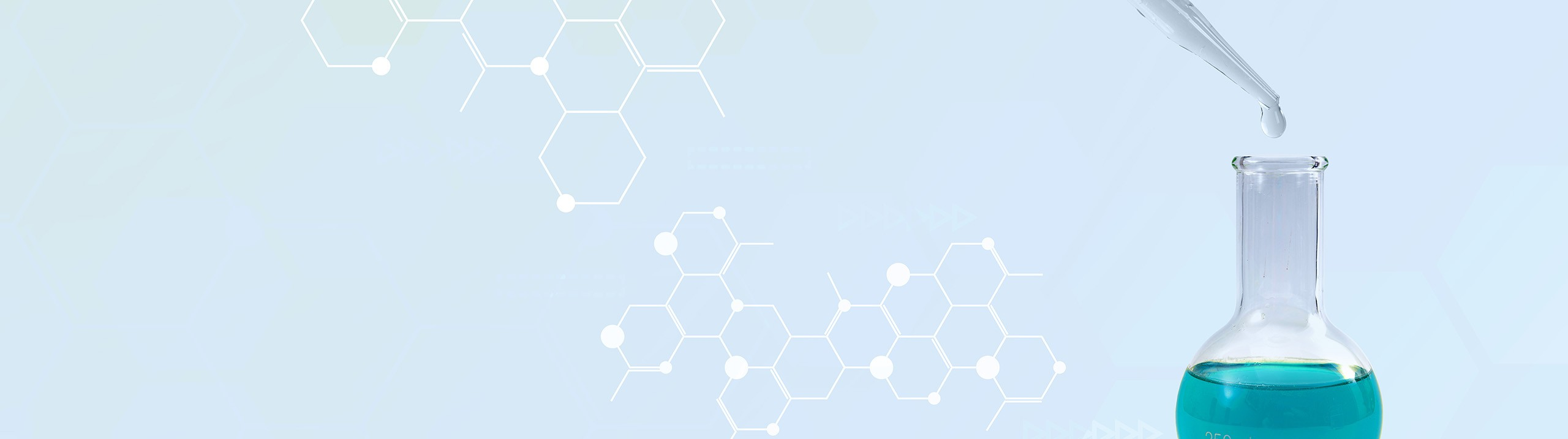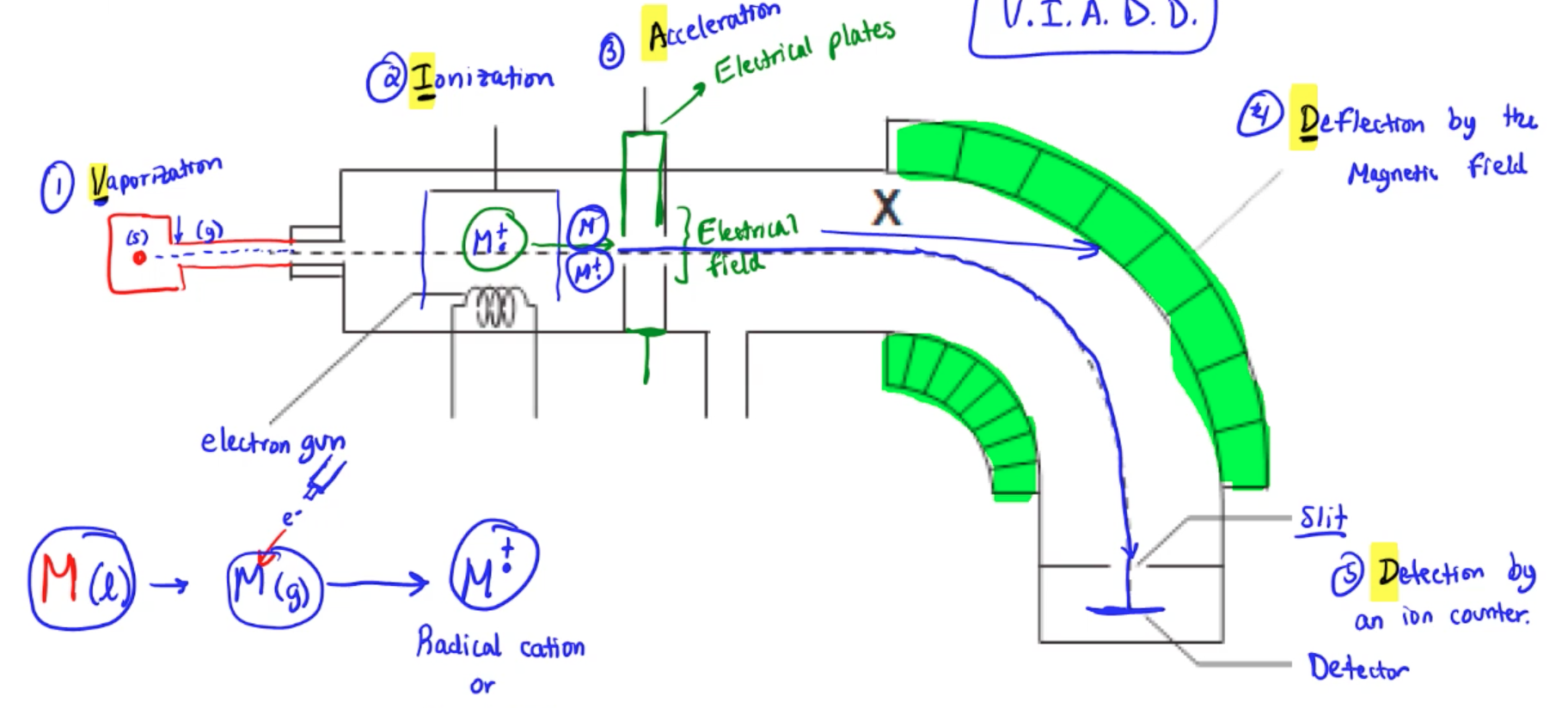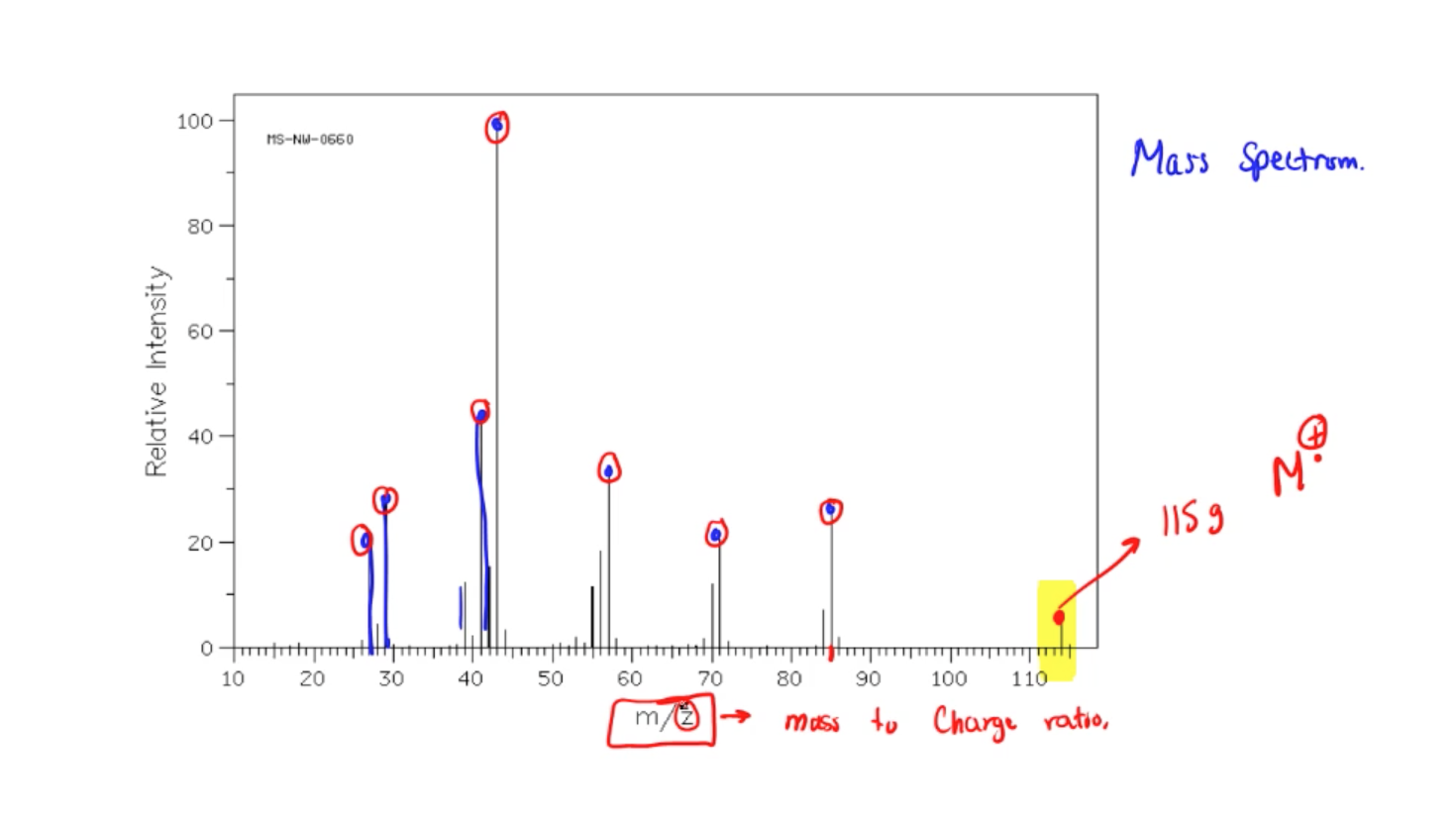## Analytical Chemistry

Learn the toughest concepts covered in your Analytical Chemistry class with step-by-step video tutorials and practice problems.

17. Fundamentals of Spectrophotometry

# Instrument Components

Mass Spectrometry
1
concept

## Mass Spectrometry8m
Play a video:
in this video, we're gonna take a look at a common mass spectrometer, Its parts and the functions that go along with it. Now we're gonna say here that when it comes to the mass spectrometer, it involves basically three fundamental things. It involves the ionization of our sample, it involves the separation of the ions created. And then finally the detection of those gasses ions. Now, the way it works is here we have the basic layout of a mass spectrometer and the way it works is we're gonna have our sample right here and now our sample will just classify and it is sample em what's gonna happen here is sample m is going to travel into the machine. So in step one we're gonna have the vaporization of our sample so it's gonna become a gas. So here it might be a liquid. But once it goes gets past this point here it can become a gaseous substance. Then we're gonna say it enters the ionization chamber here, we're gonna say here that this coil version that we see right here, This represents an electron gun which is gonna cause ionization. Okay, so step two here is represented by ionization of our gashes sample. So basically an ionization. This electron gun basically shoots out an electron and it shoots out the electron so fast that it knocks off an electron off of my sample. Em so now my sample has lost an electron so it's gonna be positive. But remember electrons come in pairs. So it's knocked off one electron. So there's now an electron that is not paired. So it's just one lone electron there we're gonna say if you have one electron that's not paired up with another electron that's referred to as a radical. So this is gonna be a radical and it has a positive charge, positive ions are called cat ions. So this represents a radical cat ion or sometimes referred to as your parent ion. Okay, so we started off as a solid here. Past this point we become gas and then at this point we have an electron knocked off. So now we represent that. We're gonna say now these this here and here. These represent electrical plates. So this thing is passing through an electrical field. So what happens here in this electrical field is acceleration because just imagine it's not one molecule of this compound, there's hundreds of them and so hundreds of them become gasses and this electron gun is shooting several electrons at a time. Some of the gasses get hit and become these radical cat ions. Some don't get hit. So it's a mixture of gas particles that have no charge with gas particles that do have charges passing through this electric field. The ones that possess a charge are the ones that are getting affected by the electric field and the electric field's gonna cause them the speed through this very fast Then when we get to this curve here, we're gonna say this portion and this portion that I have in green, these represent magnets. Now the particles that have charges are gonna be affected by the magnet. So at the fourth step we're gonna have deflection by the magnetic field. So those with charges are gonna bend because of the two magnets. This way the particles that have no charge because some of them are not gonna have charges. They're not gonna be able to be affected by the magnet. So they're just gonna crash into the wall and get destroyed. So they're gonna be lost. Then what's happening here is we're going through this slit and what they're doing here now is they're gonna get hit, they're gonna hit this last portion here. This is our detector. And so step five deals with detection. Okay, by an ion counter. Okay, so that's basically looking at the the number of electrons that are the number of ions that are hitting the detector. What are their sizes? Um what are the varieties of fragments I'm getting? And this information is linked up with a computer and being linked up to the computer provides us data and this data creates what we call this mass spectrum chart. So each one of these lines here, I know there's many of them represents basically a fragment that hits that detector and from these fragments we can piece together a representation of what we think the compound um looks like based off each one of these signals. Each one of these signals represents a fragment that hit the detector and piecing them all together can give us the complete mass of the unknown sample And in fact we look all the way on the right At the last one at the last peaks. This peak here which is looks like it's around 115 grams represents my starting material which is my parent ion. And what's happening here, it's getting broken up into pieces. And those pieces that are hitting the detector are each one of these guys here. The smaller ones could just be background noise. So we don't really look at them too much. We're looking at the higher peaks now notice here that in this graph we have what's called MZ. That EMC represents our mass to charge ratio. So basically each one of these fragments is gonna possess a positive charge. So it's their masses. Like this one here is about 85, its mass and then its charges plus one. So all of them have a mass to charge ratio. That's the whole thing about um aspect um Spectrometer. It basically breaks up our sample basically destroying it. But in the process of destroying it we're able to calculate the masses of each fragment that's hitting the detector. And in that way we can predict what our original sample weighed before we started to destroy it. This is just a way of us calculating the mass of an unknown sample. That's the whole point of using a mass spectrometer. And these are the main five things that the mass spectrometer is doing and telling us All right. So we have vaporization and step one. We have ionization, we have acceleration deflection and then finally detection. Okay, so here, just remember V I A D D. And then technically you could add one more for step six, which is record because when it's hitting the detector, it's connected to a computer. Okay. And being connected to that computer, it's what develops this mass spectrum at the end. This mass spectrum that we created here. So, if you remember each one of those letters, that will tell us all the steps when it comes to a mass spectrometer and the development of our spectrum at the end. So just remember this whole process is just to help us figure out the weight of an unknown sample.

mass spectrometer involves three primary functions:

1. The vaporization of your unknown compound M into gas M.

2. The ionization of gas M into the molecular ion or radical cation.

3. The detection of all gas ion fragments.Analysis of all gaseous ions helps to create a mass spectrum.